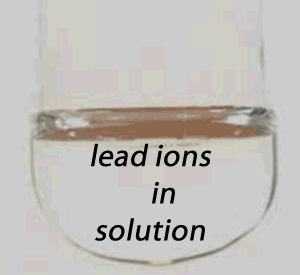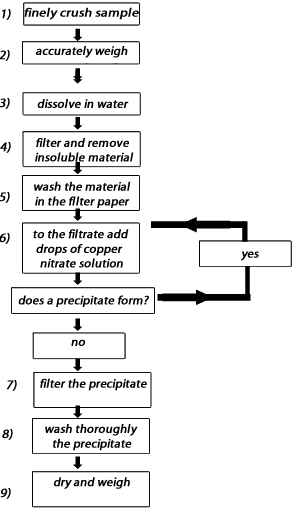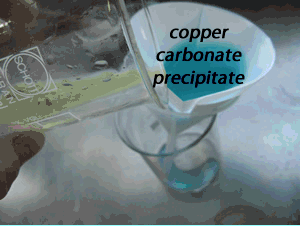Gravimetric analysisGravimetric analysis is a method by which a desired constituent of a solution is isolated, usually by precipitation, either in its pure form or in a compound. The precipitate is then weighed to ultimately determine the amount of the desired constituent. Often silver ions are used to precipitate chloride ions out of solution and therefore determine the salt content or barium ions used to precipitate sulfates and therefore determine the percentage of sulfate in fertiliser. In order to successfully undertake gravimetric analysis you should be familiar with stoichiometry, precipitates and simple mole calculations as all gravimetric problems require the calculation of the mole of precipitate as the initial step in the calculations. The best way to learn gravimetric analysis is to jump straight into some examples. Below is the first. Simply click on the blue writing to obtain answers. A student was given a sample of a carbonate and asked to identify it as one of three possible carbonates, lithium carbonate (Li2CO3) , sodium carbonate(Na2CO3) or potassium carbonate (K2CO3) 1.06 grams of the finely crushed carbonate was placed in a 300 mL beaker and 200 mL of water added and the resulting mixture filtered to remove insoluble material. To the filtrate, copper nitrate was added and a blue precipitate was formed. A flow chart of the procedure is shown on the right. The precipitate was filtered dried and weighed at 1.23 grams. Identify the carbonate. Step 1 Like with all gravimetric problems a plan of attack is essential. The difference between the three carbonates is their formula mass. Now formula mass is something that you can find if you know the mole and mass of the carbonate. Once we know the formula mass of the compound we can identify it. Step 2 The precipitate formed should be identified as copper carbonate. Write the equation for its formation. CO3-2(aq) + Cu+2(aq) => CuCO3 Step 3 Find the mol of copper carbonate that has precipitated. This works out to be 0.00996 mol. Step 4 According to the equation above for every mole of copper carbonate formed one mole of carbonate is needed. So mole of copper carbonate = mole of carbonate ions = 0.00996 Step 5 All three unknowns have the following formulae lithium carbonate (Li2CO3) , sodium carbonate(Na2CO3) potassium carbonate (K2CO3) So one mol of each carbonate contains one mol of carbonate ions hence 0.00996 mol of carbonate ions gives 0.00996 mol of carbonate compound. Step 5 Calculate the formula mass of the carbonate. This is 106.43. It must be sodium carbonate.It is important to wash the precipitate trapped in the filter paper thoroughly. Many unwanted ions may adhere to the crystals of the precipitate and if not washed off will give an inflated value of the mass of the precipitate. In this case since we are using copper nitrate to precipitate the carbonate ions the nitrate ions (NO3-) will be present on the precipitate and need to be washed off, as shown on the right1) Consider the flow chart above. a) Why is it important to wash thoroughly the insoluble material trapped in the filter paper at step 5? b) What is the impact on the formula mass of the carbonate compound if the insoluble material is not washed? c) Why is it important to wash thoroughly the precipitate trapped in the filter paper at step 8? d) What is the impact on the formula mass if the precipitate is not washed? 2) At step 6 we continue to add drops of copper nitrate to the filtrate and proceed only when there is no more precipitate to be formed. Explain why 3) Why was the sample finely crushed at step 1) in the flow chart above? Continue